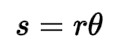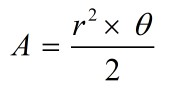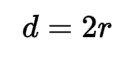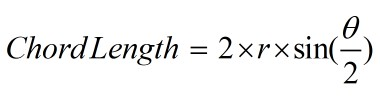Request a Tool

Arc length Calculator

Arc Length Calculator use to calculate arc length, sector area, diameter and chord d in degree and radian.

Arc Length
0
Arc Length
0

Formula• s = Arc length (in radians)
• θ = Central angle in radians

Defination / Uses

The distance between two places along a portion of a curve is known as arc length. Curve rectification is the process of determining the length of an uneven arc segment. Infinitesimal calculus gave rise to a generic formula that, in some situations, yields closed-form answers.

Sector Area
0
Sector Area
0

Formula• A = Sector area
• θ = Central angle in radians

Defination / Uses

The area of a circle's sector is the amount of space encompassed within the sector's boundary. The origin of a sector is always the circle's centre. The section of a circle encompassed by its two radii and the arc adjoining them is known as a sector of a circle.

Diameter
0
Diameter
0

Formula• d = Diameter

Defination / Uses

Any straight line segment that goes through the centre of the circle and whose endpoints are on the circle is called a diameter of a circle in geometry. It can also be described as the circle's longest chord. Both definitions are correct for a sphere's diameter.

Chord d
0
Chord d
0

Formula